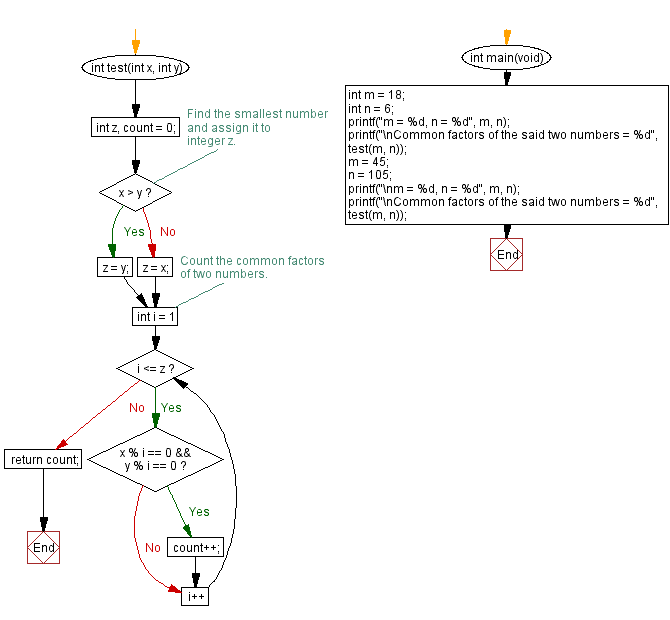﻿ C - Count common factors of two integers

# C Programming: Count common factors of two integers

## C Programming Mathematics: Exercise-36 with Solution

Write a C program to count common factors of the two given integers.

Factor - A number or algebraic expression that divides another evenly, that is, without leaving a remainder.

Example:
Input: m = 18, b = 6
Factors of 18 are 1, 2, 3, 6, 9, 18
Factors of 18 are 1, 2, 3, 6
Common factors of the said two numbers are - 1,2,3,6
Output: 4

Test Data:
(18, 6) -> 4
(45, 105) -> 4

Sample Solution:

C Code:

``````#include <stdio.h>
#include <math.h>

int test(int x, int y) {
int z, count = 0;
//Find the smallest number and assign it to integer z.
if (x > y) {
z = y;
} else {
z = x;
}
//Count the common factors of two numbers.
for (int i = 1; i <= z; i++) {
if (x % i == 0 && y % i == 0) {
count++;
}
}
return count;
}
int main(void) {
int m = 18;
int n = 6;
printf("m = %d, n = %d", m, n);
printf("\nCommon factors of the said two numbers = %d", test(m, n));
m = 45;
n = 105;
printf("\nm = %d, n = %d", m, n);
printf("\nCommon factors of the said two numbers = %d", test(m, n));
}

```
```

Sample Output:

```m = 18, n = 6
Common factors of the said two numbers = 4
m = 45, n = 105
Common factors of the said two numbers = 4
```

Flowchart:C Programming Code Editor:

Improve this sample solution and post your code through Disqus.

What is the difficulty level of this exercise?

Test your Programming skills with w3resource's quiz.

﻿